# 神器-可视化分析之Basemap实战详解（二）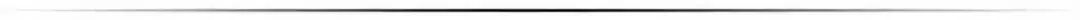### BaseMap 画世界地图

BaseMap 画世界地图，主要方法是画地球海岸线，画国家分界线主要方法是： `m.drawcoastlines()`

BaseMap 画地球地图详细代码如下：

``````import matplotlib.pyplot as plt
from mpl_toolkits.basemap import Basemap
import numpy as np

def drawcoast():
plt.figure(figsize=(12, 8))
m = Basemap()   # 创建一个地图
m.drawcoastlines()   # 画海岸线
plt.show()   # 显示图像
if __name__ == '__main__':
drawcoast()
``````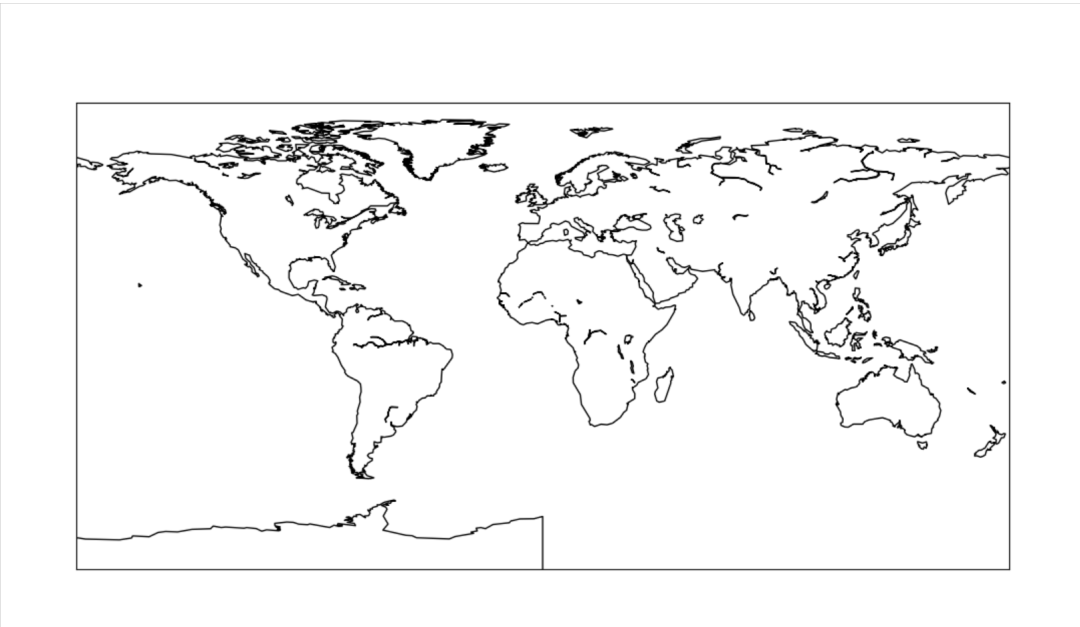### 用 BaseMap 画中国地图

``````# 画中国地图
def draw_china():
plt.figure(figsize=(10, 6))
m = Basemap(llcrnrlon=77, llcrnrlat=14, urcrnrlon=140, urcrnrlat=51, projection='lcc', lat_1=33, lat_2=45,
lon_0=100)
m.drawcountries(linewidth=1.5)
m.drawcoastlines()
plt.show()
``````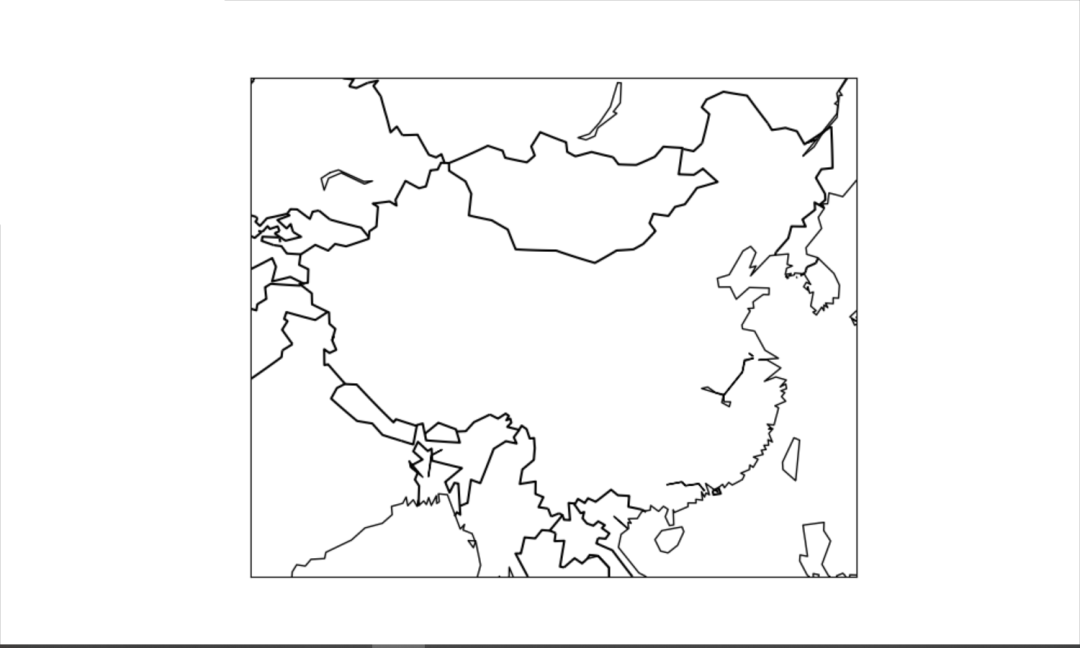### 用 BaseMap 绘制地球

``````def draw_basic():
map = Basemap(projection='ortho', lat_0=0, lon_0=0)
map.drawmapboundary(fill_color='aqua')
map.fillcontinents(color='gray',lake_color='aqua')
map.drawcoastlines()
plt.show()
``````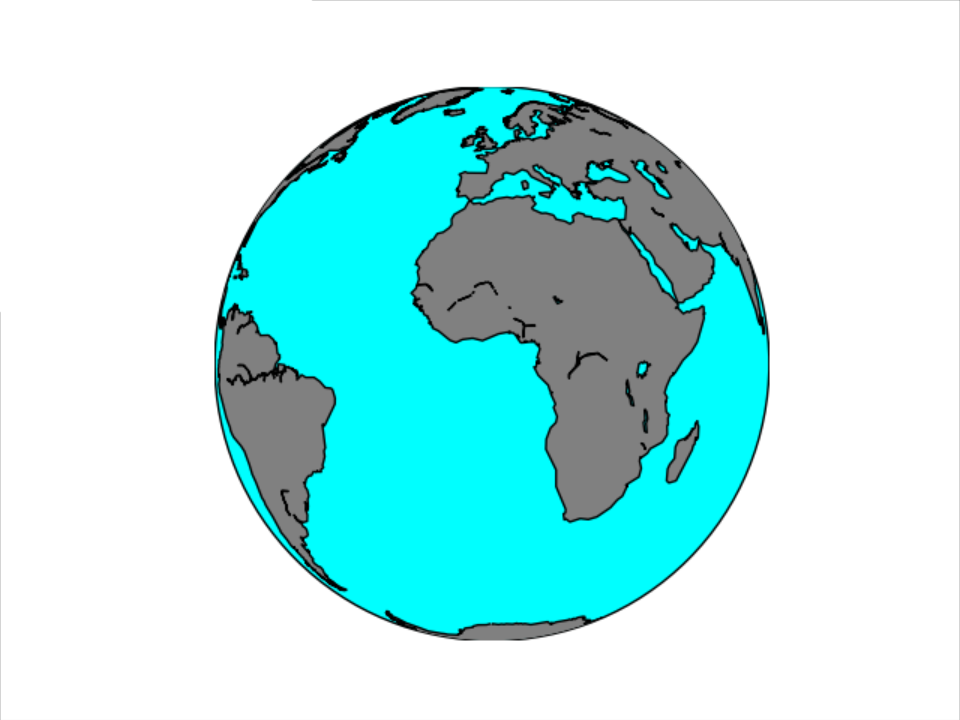### 用 BaseMap 绘制人口分布图

[En]

According to the main population distribution of each city, the urban population distribution map is drawn. The detailed method and code are as follows:

``````def drawearth():
names = []
pops = []
lats = []
lons = []
countries = []
for line in file:
info = line.split()
names.append(info)
pops.append(float(info))
lat = float(info[:-1])
if info[-1] == 'S': lat = -lat
lats.append(lat)
lon = float(info[:-1])
if info[-1] == 'W': lon = -lon + 360.0
lons.append(lon)
country = info
countries.append(country)
# set up map projection with
# use low resolution coastlines.
map = Basemap(projection='ortho', lat_0=35, lon_0=120, resolution='l')
# draw coastlines, country boundaries, fill continents.
map.drawcoastlines(linewidth=0.25)
map.drawcountries(linewidth=0.25)
# draw the edge of the map projection region (the projection limb)
map.drawmapboundary(fill_color='#689CD2')
# draw lat/lon grid lines every 30 degrees.
map.drawmeridians(np.arange(0, 360, 30))
map.drawparallels(np.arange(-90, 90, 30))
# Fill continent wit a different color
map.fillcontinents(color='#BF9E30', lake_color='#689CD2', zorder=0)
# compute native map projection coordinates of lat/lon grid.
x, y = map(lons, lats)
max_pop = max(pops)
# Plot each city in a loop.
# Set some parameters
size_factor = 80.0
y_offset = 15.0
rotation = 30
for i, j, k, name in zip(x, y, pops, names):
size = size_factor * k / max_pop
cs = map.scatter(i, j, s=size, marker='o', color='#FF5600')
plt.text(i, j + y_offset, name, rotation=rotation, fontsize=10)

plt.title('earth')
plt.show()

if __name__ == '__main__':
drawearth()
``````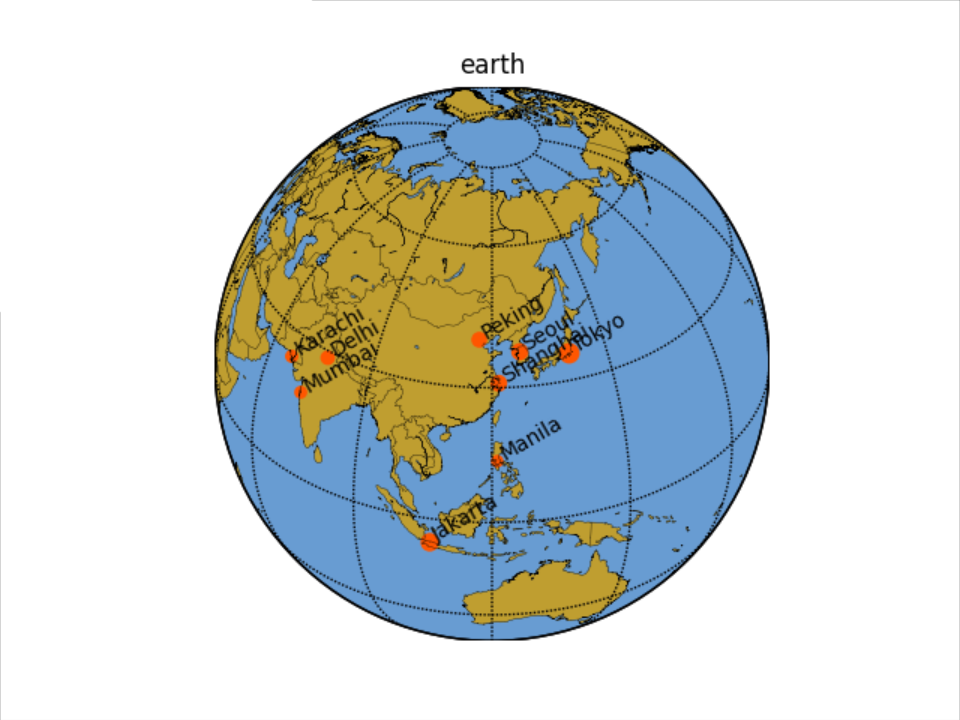### 画投影地球

``````def draw_earth1():
import matplotlib.pyplot as plt
from mpl_toolkits.basemap import Basemap
plt.figure(figsize=(8, 8))
# 正射投影，投影原点设在了上海周边
m = Basemap(projection='ortho', resolution=None, lat_0=30, lon_0=120)
# 图像原始分辨率是5400*2700，设置scale = 0.5以后分辨率为2700*1350,如此作图
# 迅速不少也不那么占用内存了
m.bluemarble(scale=0.5)
plt.show()

if __name__ == '__main__':
draw_earth1()
``````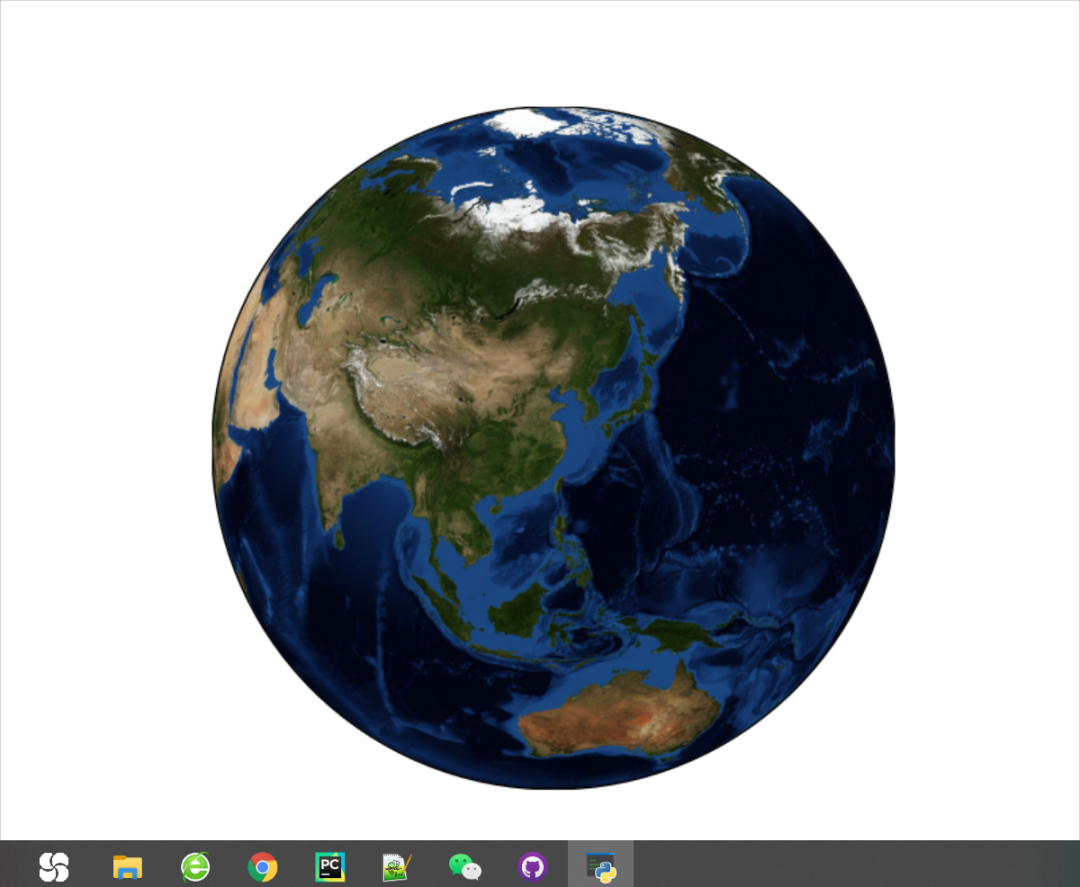### 总结

PS：公号内回复「Python」即可进入Python 新手学习交流群，一起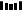Original: https://blog.csdn.net/weixin_48923393/article/details/121623672
Author: Python 技术
Title: 神器-可视化分析之Basemap实战详解（二）

## 【计算机视觉40例】案例10：隐身术

【 导读】本文是专栏《计算机视觉40 例简介》的第10 个案例《隐身术》。该专栏简要介绍李立宗主编《计算机视觉40 例——从入门到深度学习（OpenCV-Python ）》一书的40 个案例。 目前，...